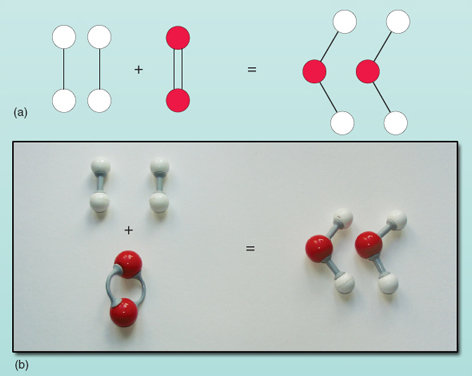# What is a Balanced Chemical Equation? Why should chemical equations be balanced?The process of making the numbers of different types of atoms equal on both sides of an equation is called balancing of equations. When the Numbers of atoms of different elements on reactant and products side are equal, then the chemical equation is called Balanced chemical equation.

For example

H2 + O2—– H2O

In this reaction H2 and O2 are reactants where H20 is the product.

Count the number of hydrogen atoms and oxygen atom in reactant and product

Number of hydrogen atoms in reactants is 2 and in product is 2

Number of oxygen atoms in reactants is 2 and in product is 1

The number of hydrogen atoms is equal on both sides, but the number of oxygen  atoms are unequal. To have two oxygen atom on right side we multiply H2O and write 2 H2O

2H2 + O2 —–2H2O

### It is important to balance a chemical equation for following reasons

• To validate the law of conservation of mass which states that the mass of the reactants should be equal to the mass of the products. The total mass of a system is thus conserved.
• This law holds true only if the number of atoms of reactants reacting together is equal to the number of product atoms formed.
• The balance chemical Equation tells us about the physical state of the reactants and products whether they are solid, liquid, gas, or aqueous.
• It tells us about heat changes that can take place in a chemical reaction. Is the symbol of heat. Hence, it is endothermic or exothermic can we deduced from a balanced chemical equation.